## ISC Computer Science Previous Year Question Paper 2014 Solved for Class 12

Maximum Marks: 70
Time allowed: 3 hours

Part – I

While answering questions in this Part, indicate briefly your working and reasoning, wherever required.

Question 1.
(a) From the logic circuit diagram given below, find the output ‘F’ and simplify it. 
Also, state the law represented by the logic diagram.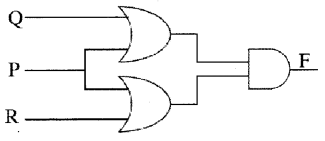(b) Write the truth table for a 2-input conjunction and disjunction in a proposition. 
(c) Find the complement of XY’Z + XY + YZ’ 
(d) Convert the following expression into its canonical POS form: 
F(A, B) = (A + B).A’
(e) Minimize the following Boolean expression using the Karnaugh map: 
$$\mathrm{F}(\mathrm{A}, \mathrm{B}, \mathrm{C})=\overline{\mathrm{A}} \mathrm{B} \overline{\mathrm{C}}+\overline{\mathrm{A}} \mathrm{BC}+\mathrm{AB} \overline{\mathrm{C}}+\mathrm{ABC}$$Question 2.
(a) State two advantages of using the concept of inheritance in Java. 
(b) An array AR [-4…. 6, -2 ….. 12 ], stores elements in Row Major Wise, with the address AR  as 4142 . If each element requires 2 bytes of storage, find the Base address. 
(c) State the sequence of traversing a binary tree in: 
(i) preorder
(ii) postorder
(d) Convert the following infix expression into its postfix form: 
(A/B + C) * (D/(E – F))
(e) State the difference between the functions int nextlnt() and boolean hasNextInt(). 
(a) (i) Existing code can be reused and functionality can be extended.
(ii) New members to the derived class can be added.
(iii) Implementation of existing methods can be replaced by overriding a method that already exists in the base class.

(b) AR [-4,… 6, -2 … 12]
Row- wise order,
AR  = B + W(n(i-1)+(j-1))
Given i = 2, j = 3, W = 2 bytes
B = ?
n = Uc – Lc + 1
= 12 – (-2) + 1
= 12 + 2 + 1
= 15
Now, 414 = B + 2 [15 (2 -(-2)) + (3-(-4))]
B + 2[60 + 7] = 4142
B + 134 = 4142 or B = 4008

(c) (i) Preorder is Root, Left, Right
(ii) Postorder is Left, Right, Root

(d) (A/B + C) * (D/(E -F))
= (A/B + C) (D/(E-F))*
= (AB/+C) (D/(EF-))*
= (AB/C+) (DEF-/)*
= AB/C + DEF-/*

(e) nextlnt() returns an integer present in a Scanner class object whereas hasNextInt() checks whether Scanner class object contains an integer or not and returns true if it contains an integer, otherwise false.

Question 3.
(a) The following functions are part of some class:

void fun 1 (char s[ ],int x)
{
System.out.println(s);
char temp;
if(x<s.length/2)
{
temp=s[x];
s[x]=s[s.length-x-1];
s[s.length-x-1 ]=temp;
fun1(s, x+1);
}
}
void fun2(String n)
{
char c[ ]=new char[n.length()];
for(int i=0;i<c.length; i++)
c[i]=n.charAt(i);
fun1(c,0);
}

(i) What will be the output of fun1() when the value of s[ ]={‘J’,‘U’,‘N’,‘E’} and x = 1? 
(ii) What will be the output of fun2( ) when the value of n = ‘SCROLL”?
(iii) State in one line what does the function fim1() do apart from recursion. 
(b) The following is a function of some class which sorts an integer array a[ ] in ascending order using selection sort technique. There are some places in the code marked by ?1?, ?2?, ?3?, ?4?, ?5? which may be replaced by a statement/expression so that the function works properly:

void selectsort(int [ ]a)
{
int i, j, t, min, minpos;
for(i=0;i {
min=a[i];
minpos = i;
for(j=?2?;y<a.length;j++) { if(min>a[j])
{
?3?=j;
min = ?4?;
}
}
t=a[minpos];
a[minpos]=a[i];
a[i]= ?5?;
}
for(int k=0;k<a.length;k++)
System. out.println(a[k]);
}

(i) What is the expression or statement at ?1? 
(ii) What is the expression or statement at ?2? 
(in) What is the expression or statement at ?3? 
(iv) What is the expression or statement at ?4? 
(v) What is the expression or statement at ?5? 
(a) (i) JUNE
JNUE
(ii) SCROLL
LCROLS
LLROCS
LLORCS
(iii) Reverses the part of a string from a specified location.
(b) (i) a. length
(ii) i + 1
(iii) minpos=j
(iv) min = a [j]
(v) t

Part – II

Answer seven questions in this part, choosing three questions from Section -A, two from Section-B and two from Section-C.

Section – A

Question 4.
(a) Given the Boolean function F(A, B, C, D) = Σ (0, 1, 2, 3, 5, 6, 7, 10, 13, 14, 15)
(i) Reduce the above expression by using, 4-variable Karnaugh map, showing the various groups (i.e., octal, quads and pairs). 
(ii) Draw the logic gate diagram for the reduced expression. Assume that the variables and their complements are available as inputs. 
(b) Given the Boolean function P(A, B, C, D) = Π (0, 1, 2, 3, 5, 6, 7, 10, 13, 14, 15)
(i) Reduce the above expression by using the 4-variable Karnaugh map, showing the various groups (i.e., octal, quads and pairs). 
(ii) Draw the logic gate diagram for the reduced expression. Assume that the variables and their complements are available as inputs. 
(a) (i) F(A, B, C, D) = Σ (0, 1, 2, 3, 5, 6, 7, 10, 13, 14, 15)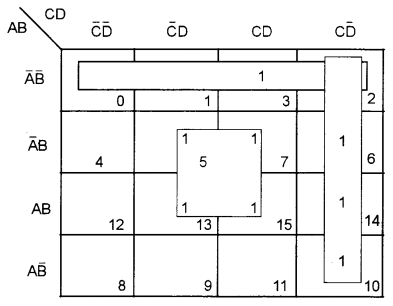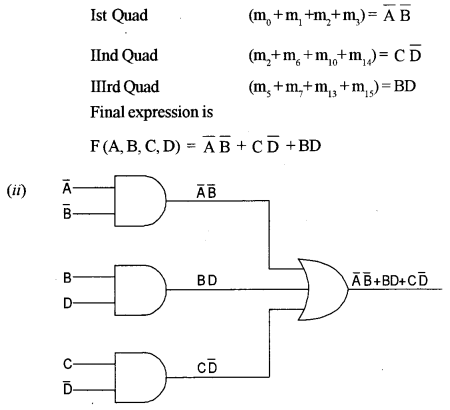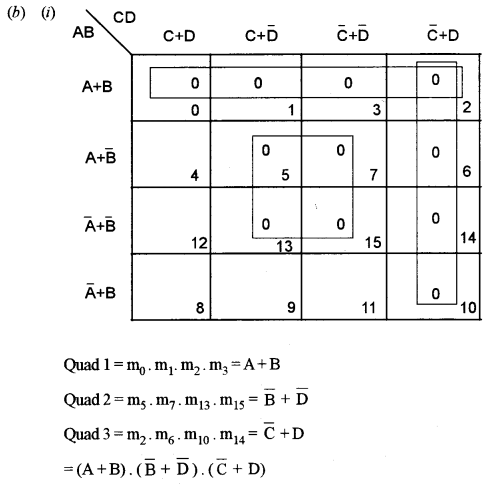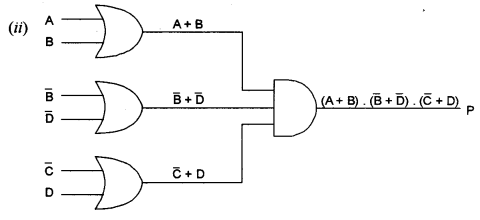Question 5.
A school intends to select candidates for the Inter-School Athletic Meet, as per the criteria are given below:
The candidate is from the Senior School and has participated in an Inter-School Athletic Meet earlier.
OR
The candidate is not from the Senior School, but the height is between 5 ft. and 6 ft. and weight is between 50 kg. and 60 kg.
OR
The candidate is from the Senior School and has a height between 5 ft. and 6 ft., but the weight is not between 50 kg. and 60 kg.
The inputs are:

 Inputs S A student is from the Senior School W Weight is between 50 kg. and 60 kg. H Height is between 5 ft. and 6 ft. A Taken part in Inter-School Athletic Meet earlier

(In all of the above cases, 1 indicates yes and 0 indicates no)
Output: X – Denotes the selection criteria [1 indicates selected and 0 indicates rejected in all cases]
(a) Draw the truth table for the inputs and outputs given above and write the SOP expression for X (S, W, H, A). 
(b) Reduce X(S, W, H, A) using Karnaugh map. 
Draw the logic gate diagram for the reduced SOP expression for X(S, W, H, A) using AND and OR gate. You may use gates with two or more inputs. Assume that the variable and their complements are available as inputs.
(a) The truth table for given input and output is: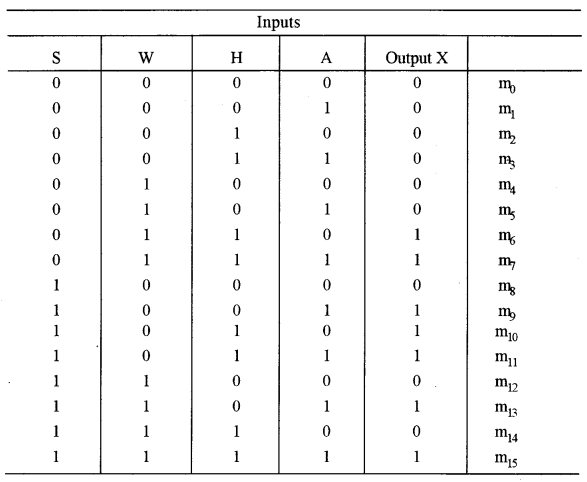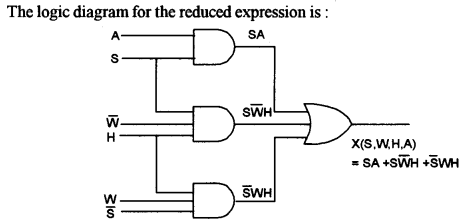Question 6.
(a) With the help of a logic diagram and a truth table, explain a Decimal to Binary encoder. 
(b) Derive a Boolean expression for the logic diagram given below and simplify it.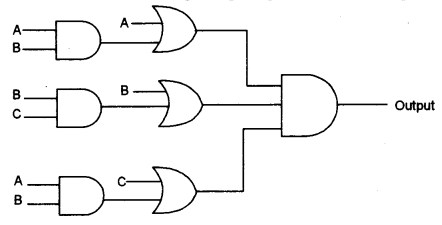(c) Reduce the following expression using Boolean laws: 
F (A, B, C, D) = (A’ + C) (A’ + C’) (A’ + B + C’ D)
(a) Decimal to binary encoder is used to convert decimal numbers into its equivalent binary form.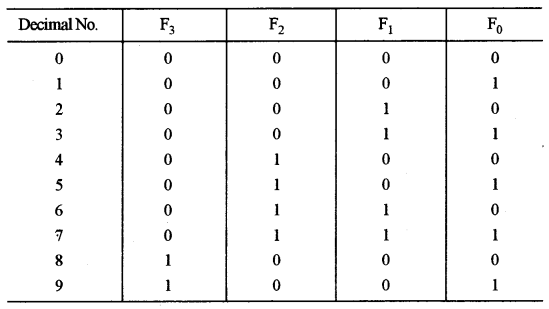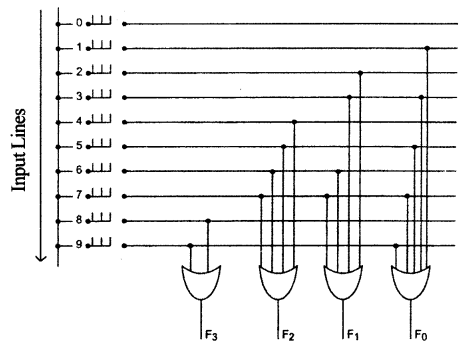Question 7.
(a) Differentiate between XNOR and XOR gates. Draw the truth table and logic diagrams of 3 input XNOR gate. 
(b) Differentiate between a proposition and wff. 
(c) Define Half Adder. Construct the truth table and a logic diagram of a HalfAdder. 
(a) XOR gate produces output as 1 when odd no. of inputs are 1 otherwise it gives 0. Whereas XNOR gate produces output as 1 when even no. of inputs are 1 otherwise it returns 0.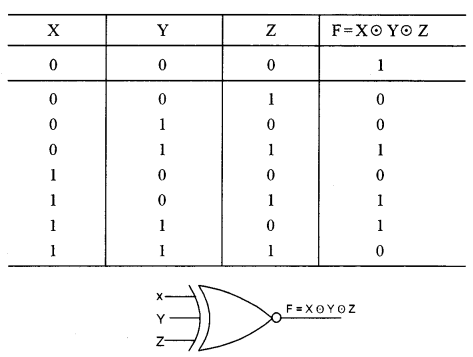(b) A proposition is an elementary atomic sentence that returns either true or false. Whereas wff’s are a well-formed formula which contains propositions and connectives.

(c) It is a digital electronic circuit used to add two bits.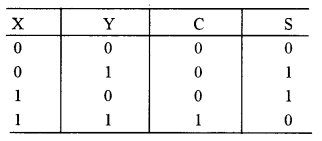Section – B

• Each program should be written in such a way that it clearly depicts the logic of the problem.
• This can be achieved by using mnemonic names and comments in the program.
• Flowcharts and algorithms are not required.
• The programs must be written in Java.

Question 8.
A class Mixer has been defined to merge two sorted integer arrays in ascending order. Some of the members of the class are given below: 
Class name: Mixer
Data members/instance variables:
int arr[ ]: to store the elements of an array
int n: to store the size of the array
Member functions:
Mixer(int nn): constructor to assign n=nn
void accept(): to accept the elements of the array in ascending order without any duplicates
Mixer mix (Mixer A): to merge the current object array elements with the parameterized array elements and return the resultant object
void display(): to display the elements of the array
Specify the class Mixer, giving details of the constructor(int), void accept(), Mixer mix(Mixer) and void display(). Define the main( ) function to create an object and call the function accordingly to enable the task.

import java.util.*;
class Mixer
{
intarr[];
int n;
static Scanner sc=new Scanner(System.in);
Mixer(int nn)
{
n=nn;
arr=new int[n];
}
void accept()
{
System.out.println("Enter"+ n+ " elements in ascending order");
for(int i=0; i<n; i++)
arr[i]=sc.nextInt();
}
Mixer mix(Mixer A)
{
Mixer B=new Mixer(n+A.n);
int x=0, y=0, z=0;
while(xA.arr[y])
{
B.arr[z]=A.arr[y];
y++;
}
else
{
B.arr[y]=arr[x];
x++;
}
z++;
}
while(x<n)
B.arr[z++]=arr[x++];
while(y<A.n)
B.arr[z++]=A.arr[y++];
return B;
}
void display()
{
for(int i=0;i<n;i++)
System.out.println(arr[i]);
}
static void main()
R=P.mix(Q);
R. display();
}
}

Question 9.
A class SeriesSum is designed to calculate the sum of the following series:
$$\mathrm{Sum}=\frac{\mathrm{x}^{2}}{1 !}+\frac{\mathrm{x}^{4}}{3 !}+\frac{\mathrm{x}^{6}}{5 !}+\ldots \frac{\mathrm{x}^{\mathrm{n}}}{(\mathrm{n}-1) !}$$
Some of the members of the class are given below:
Class name: SeriesSum
Data members/instance variables:
x: to store an integer number
n: to store the number of terms
sum: double variable to store the sum of the series
Member functions:
SeriesSum(int xx, int nn): constructor to assign x=xx and n=nn
double find fact(int m): to return the factorial of m using the recursive technique.
double find power(int x, int y): to return x raised to the power of y using the recursive technique.
void calculate(): to calculate the sum of the series by invoking the recursive functions respectively
void display(): to display the sum of the series
(a) Specify the class SeriesSum, giving details of the constructor(int, int), double find fact(int),
double find power(int, int), void calculate() and void display(). Define the main() function to create an object and call the functions accordingly to enable the task. 
(b) State the two differences between iteration and recursion. 

(a) class SeriesSum
{
int x, n;
double sum;
SeriesSum(int xx, int nn)
{
x=xx;
n=nn;
sum=0.0;
}
double findfact(int a)
{
return (a<2)? 1:a*findfact(a-1);
}
double findpower(int a, int b)
{
return (b==0)? 1:a*findpower(a,b-1);
}
void calculate()
{
for(int i=2;i<=n;i+=2)
sum+= findpower(x, i)/findfact(i-1);
}
void display()
{
System.out.println("sum="+ sum);
}
static void main()
{
SeriesSum obj = new SeriesSum(3, 8);
obj.calculate();
obj.display!);
}
}

(b) Iteration: Fast process and less memory.
Recursion: Slow process and more memory.

Question 10.
A sequence of Fibonacci strings is generated as follows:
S0 = “a”, SF = “b”, Sn = S(n-1) + S(n-2) where ‘+’ denotes concatenation. Thus the sequence is:
a, b, ba, bab, babba, babbabab,……. n terms. 
Design a class FiboString to generate Fibonacci strings. Some of the members of the class are given below:
Class name: FiboString
Data members/instance variables:
x: to store the first string
y: to store the second string
z: to store the concatenation of the previous two strings
n: to store the number of terms
Member functions/methods:
FiboString(): constructor to assign x=”a”, y=”b” and z=”ba”
void accept(): to accept the number of terms ‘n’
void generate(): to generate and print the Fibonacci strings. The sum of (‘+’ ie concatenation) first two strings is the third string. Eg. “a” is first string, “b” is second string then the third will be “ba”, and fourth will be “bab” and so on.
Specify the class FiboString, giving details of the constructor(), void accept() and void generate(). Define the main() function to create an object and call the functions accordingly to enable the task.

importjava.util.*;
class FiboString
{
String x,y,z;
int n;
FiboString()
{
x="a";
y="b";
z="ba";
}
void accept()
{
Scanner Sc = new Scanner (System.in);
System. out.println ("Enter number of terms");
n = Sc.nextInt();
}
void generate()
{
System. out.print(x+","+y);
for(int i=0; i<=n-2; i++)
{
System.out.print(","+z);
x=y;
y=z;
z=y+x; OR z= y.concat(x);
}
}
static void main()
{ FiboString obj=new FiboString();
obj.accept();
obj.generate();
}
}

Section – C

• Each program should be written in such a way that it clearly depicts the logic of the problem stepwise.
• This can be achieved by using comments in the program and mnemonic names or pseudo-codes for algorithms.
• The programs must be written in Java and the algorithms must be written in general/standard form, wherever required/specified.
• Flowcharts are not required.

Question 11.
A superclass Stock has been defined to store the details of the stock of a retail store. Define a subclass Purchase to store the details of the items purchased with the new rate and updates the stock. Some of the members of the classes are given below: 
Class name: Stock
Data members/instance variables:
item: to store the name of the item
qt: to store the quantity of an item in stock
rate: to store the unit price of an item
amt: to store the net value of the item in stock
Member functions:
Stock (…): parameterized constructor to assign values to the data members
void display(): to display the stock details
Class name: Purchase
Data members/instance variables:
pqty: to store the purchased quantity
prate: to store the unit price of the purchased item
Member functions/ methods:
Purchase(…): parameterized constructor to assign values to the data members of both classes
void update (): to update stock by adding the previous quantity by the purchased quantity and replace the rate of the item if there is a difference in the purchase rate. Also, update the current stock value as (quantity * unit price)
void display(): to display the stock details before and after updation.
Specify the class Stock, giving details of the constructor() and void display(). Using the concept of inheritance, specify the class Purchase, giving details of the constructor(), void update() and void display().
The main function and algorithm need not be written.

class Stock
{
String item; doubleqty,rate,amt;
Stock(String a, double b, double c)
{
item=a;
qty=b;
rate=c;
amt=qty * rate;
}
void display()
{
System.out.println("Name of the item: "+item);
System.out.println("Quantity: "+qty);
System.out.println("Rate per unit: "+rate);
System.out.println("Net value: "+amt);
}
}
class Purchase extends Stock
{
int pqty;
double prate;
Purchase(String a, double b, double c, int d, double e)
{
super(a, b, c);
pqty=d;
prate=e;
}
void update()
{
qty += pqty;
if(prate!=rate)
rate=prate;
amt = qty * rate;
}
void display()
{
super.display();
update();
super.display();
}
}

Question 12.
A stack is a linear data structure which enables the user to add and remove integers from one end only, using the concept of LIFO(Last In First Out). An array containing the marks of 50 students in ascending order is to be pushed into the stack.
Define a class Array_to_Stack with the following details: 
Class name: Array to Stack
Data members/instance variables:
m[]: to store the marks
st[ ]: to store the stack elements
cap: maximum capacity of the array and stack
top: to point the index of the topmost element of the stack
Methods/Member functions:
Array_to_Stack(int n): parameterized constructor to initialize cap = n and top = -1
void input_marks(): to input the marks from the user and store it in the array m[ ] in ascending order and simultaneously push the marks into the stack st[ ] by invoking the function pushmarks()
void pushmarks(int v): to push the marks into the stack at top location if possible, otherwise, display “not possible”
intpopmarks(): to return marks from the stack if possible, otherwise, return-999
void display(): To display the stack elements
Specify the class Array_to_Stack, giving the details of the constructor(int), void input_marks(), void pushmarks(int), int popmarks() and void display().
The main function and the algorithm need not be written.

importjava.util.*;
class Array to Stack
{
int m[], stD;
int cap, top;
static Scanner sc=new Scanner(System.in);
Array_to_Stack(int n)
{
cap = n;
top = -1;
m=newint[cap];
st=new int[cap];
}
void input_marks()
{
System.out.println("Enter "+cap+" elements in ascending order");
for(int i=0;i<cap;i++)
{
m[i]=sc.nextInt();
pushmarks(m[i]);
}
}
void pushmarks(int v)
{
if (top<cap-1)
st[++top]=v;
else System.out.println("stack is full");
}
int popmarks()
{
if(top>=0)
retumst[top--];
else
return-999;
}
void display()
{
for(int i=top;i>=0 ;i--)
System.out.println(st[i]);
}
}

Question 13.
(a) A linked list is formed from the objects of the class: 

class Node
{
int number,
Node nextNode;
}

Write an Algorithm OR a Method to add a node at the end of an existing linked list. The method declaration is as follows:
void add node (Node start, int num)
(b) Define the terms complexity and big-O notation.
(c) Answer the following from the diagram of the Binary Tree given below: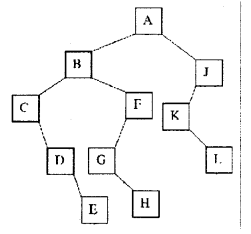(i) The root of the tree. 
(ii) Left subtree 
(iii) Inorder traversal of the tree 
(iv) Size of the tree. 
(a) Algorithm to add a node at the end of an existing linked list.
Steps:

1. Start
2. Set temporary pointer to start node
3. Repeat steps 4 until it reaches null
4. move the pointer to the next node
5. create a new node, assign num to number and link to the temporary node
6. End

Method to add a node at the end of an existing linked list.

void add node (node start, int num)
{
Node A = new Node(start)
while (A != null)
A=A.nextNode;
Node C = new node ();
C. number = num;
C.nextNode = null;
A. next = C;
}

(b) Complexity: It is the measurement or growth rate of an algorithm with respect to time and space.
Big ‘O’ notation: It is a unit of measurement of an algorithm or represents complexity.
(c) (i) A
(ii) B, C, F, D, G, E, H
(iii) C, D, E, B, G, H, F, A, K, L, J
(iv) 11